# Python: The Super Champ for Machine Learning

0
496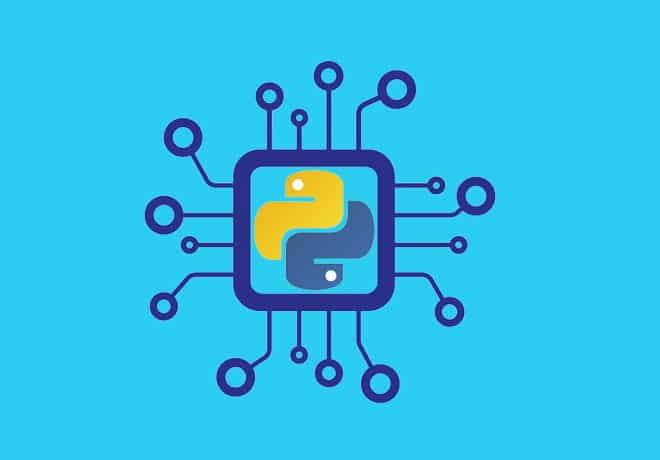Machine Learning (ML) is a technology used to make any software process improve through experience with the help of algorithms. It is a part of artificial intelligence (AI) and uses training data to improve decisions and predictions, without the need for any explicit programming. ML was first conceived by Arthur Samuel of IBM in 1952. This article highlights Python as the best language for ML.

In business, ML is used to resolve various problems and the process is referred to as predictive analysis. To run any static process, it’s possible to program a computer using specific algorithms. However, the computer must modify its program and improve the algorithm to handle dynamic processes in real-time. Arthur Samuel of IBM created the world’s first ML program to run checkers video games on IBM 701 computers in 1952. There are mainly two objectives of ML — one is to classify data models, which have been developed using I/O data, and the other is to predict future outputs based on that data. These two objectives can be achieved using various learning algorithms, and also with the help of a powerful programming language like Python.

Types of learning approaches
In ML, an agent can be made intelligent using various learning approaches and algorithms. In such a learning process, ML has to go through three stages – training, validation and testing. This is done in order to process the input data and modify the algorithm for improving prediction and performance. There are various types of learning approaches available, but the three main traditionally used approaches are supervised, unsupervised and reinforcement learning.

Supervised learning
As the name suggests, this learning approach involves training the agent by a supervisor, which is usually the user. Here, the training is given to the agent using a set of training data, as examples or data models. The data contains a label and a feature. The set of data also contains the mapping of the input and output data based on the data model. So, when any new data is fed into the agent as input data, it also generates new output data based on the data model. A simple example of a data model would be a table containing rows and columns, which has data about various laptops with different specifications and price tags. So, by looking at the table one can understand which laptops are expensive and which are cheap. If this model is used for ML training, then the agent would be able to predict if a laptop with higher CPU speed will cost more or less.

The accuracy and method of processing the data depends on the algorithm and the training data used for the ML. If the output data is not satisfactory, then modification is done to the algorithm and the training data by the supervisor. Then the agent is fed again with new data and the output is collected (as a feedback to the supervisor). In case the output is not satisfactory, more modifications are done and the cycle continues until the agent is stable and matured. Here, the Naive Bayes algorithm is used because it’s best known for its ability to classify and predict data.

The Naive Bayes algorithm works on the following formula: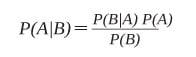, where:
A, B = events
P(A|B) = probability of A given B is true
P(B|A) = probability of B given A is true
P(A), P(B) = the independent probabilities of A and B
However, if you need to understand the above formula in simple English, then it is: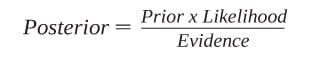The Posterior probability for supervised training would be the expected prediction data. To get that data, the Prior probability data is always updated. You can say that it’s like history data. Likelihood is the data that you can assume from previous events, but Evidence is the data that is already known and correct.

Example: If the chance of getting attacked by a dangerous computer virus is 1 Per cent but the detection of any virus by cybersecurity software is 10 Per cent, and 90 Per cent of viruses are not harmful, then the probability of getting any harm from a dangerous virus would be: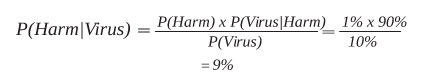The algorithm is:
1. Separate the training data by class.
2. Summarise data sets by finding the mean and standard deviation using the formula:
Mean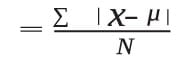, x is the feature data and N is the number of feature data.
µ = (x1 + x2 + x3… + xn)/N

Standard deviation =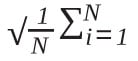(xi – µ)2, xi is the featured data starting with the index value of i.
3. Summarise data by class.
4. Calculate the probability using the Gaussian Probability Density Function
formula: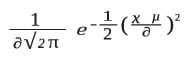, where µ is the mean and д is the standard deviation.
5. Predict class probability using the equation:

`P(Class|dataset) = P(X|Class) × P (Class)`

…which is a simplified version of the Naive Bayes formula.

Unsupervised learning
This learning approach requires no supervisor and the agent uses unlabelled data for input processing. The algorithm used in this learning approach helps the agent to find structure in its input, so that it can group or cluster data based on its patterns. Here, the input data is clustered by the agent accordingly, and can also discover hidden data. After clustering the data, the agent tries to find similarities between them and creates a relationship model. This learning requires a proper learning environment and the better the input data, the better is the learning. The algorithm used in this learning is called K-Means and the formula is: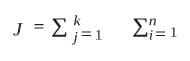|| xi(j) – cj ||2

Here,

• J = objective function
• k = number of clusters
• n = number of cases
• xi(j) = case i
• cj = centroid for cluster j
• xi(j) – cj = distance function

Clustering can also be done using a simple Euclidean distance formula:

√ (xx – x1)2 + (xy – y1)2

Here, Xx and Xy are observed values and x1 and y1 are actual/centroid values.
Example: You can divide the following data set into two clusters:

 Data ID X Y 1 1 2 2 3 4 3 5 6 4 7 8 5 9 10

First you find the centroids and create the two clusters (C1, C2) by taking the values of two Data IDs (1,2):

 Clusters X Y Centroid C1 1 2 (1,2) C2 3 4 (3,4)

Now you find in which cluster (C1 or C2) the next values of Data ID (3) will be included by using the Euclidean distance formula as follows:

```C1 = √(5–1)<sup>2</sup> + (6 – 2)<sup>2</sup>
= √(4)<sup>2</sup> + (4)<sup>2</sup>
= √32 = 5.65
C2 = √(5–3)<sup>2</sup> + (6–4)<sup>2</sup>
= √(2)<sup>2</sup> + (2)<sup>2</sup>
= √8 = 2.28```

Now, as the value of C2 is less than C1, the Data ID (3) will be clustered with C2.
Next, the new centroid values of C2 are:

```C2 = ((X<sub>c2</sub> + X<sub>3</sub>)/2,(Y<sub>c2</sub> + Y<sub>3</sub>)/2)
= ((3+5)/2,(4+6)/2)
= 4,5```

Similarly, the values for the next Data IDs can be found by using the new centroids of C2 and carrying on the clustering process.

The algorithm is:
1. Cluster data into k groups, where k is predefined.
2. Select random cluster centroids from the k points.
3. Allocate data to the nearest clusters according to the Euclidean distance calculations.
4. Calculate new cluster centroids for next data.
5. Repeat steps from 2 to 4 until no further clustering is possible.

Reinforcement learning
This type of learning works based on a reward/penalty policy and the agent is programmed to do certain predefined functions in an environment. After executing the functions, the agent gets feedback from the environment, either as a reward or penalty. This changes the state of the environment and the agent also gathers the state of the environment as an input. Depending on the state and reward values, the agent takes decisions. Depending on the rewards and state changes, the agent improves its quality of performance. This type of learning approach is used in service robots to train them in certain environments and it doesn’t require any training data sets or data models. Here, the Q-learning algorithm is used and works with the following formula:

Qnew (st, at) ← Qold(st, at) + α.(rt + y. maxaQ(st+1, a) – Qold (st, at))

Here,
Qnew is the new state of the agent
st is the state of the agent in time t
at is the action taken by the agent in time t.
α is the learning rate
rt is the reward in time t
γ is the discount factor

Example: If the agent takes actions (A1,A2,A3,A4), causes state changes (S1,S2,S3,S4) and receives a set of rewards R, then the Q values will be initialised and the rewards data will be created as follows:

 Q = States A1 A2 A3 A4 S1 0 0 0 0 S2 0 0 0 0 S3 0 0 0 0 S4 0 0 0 0

 R = States A1 A2 A3 A4 S1 0 0 1 S2 0 0 0 S3 0 0 1 S4 0 0 1

Here, the reward values are either 1 or 0 based on the actions.

If the agent is in state S4, then there are three possible actions that can change the state to S1, S3, and S4. This will be calculated using a simplified formula:

```Q(sate, action) = R(state, action) + y × Max[Q(next state, all actions)]
Q(S1, A4) = R(S1, A4) + 0.8 × Max[Q(S4, A1),( S4, A3),( S4, A4)]
= 1 + 0.8 × 0 = 1```

Here, the value of Max[Q(next state, all actions)] is zero, because the Q values were initialised to zero.

So, as the new Q value for Q(S1, A4) is 1, the Q values will be updated:

 Q = States A1 A2 A3 A4 S1 0 0 0 1 S2 0 0 0 0 S3 0 0 0 0 S4 0 0 0 0

Similarly, the next states are determined by the agent, and the Q values will keep on updating.

The algorithm is:
1. Agent starts in state (st) and Q values are initialised.
2. Take action at and wait for a reward (rt) and state (st) change.
3. Update the reward (rt) and state (st) values.
4. Calculate the next action using the Q-learning formula.
5. Update Q values.

Among the three learning approaches, supervised learning is used only when both the input and output data is available along with the data model. Unsupervised learning is used only when the input data is available, and reinforcement learning is required when there is no training data and the agent is required to be trained from the working environment. Apart from these, there are many other learning approaches like self-learning, feature learning, sparse dictionary learning, anomaly detection, robot learning, and association rule learning.

Hardware and software for ML
To run any computer program (algorithm) a central processing unit (CPU) is required. Most of the CPU hardware contains multiple cores, but such CPUs are designed for serial operations and don’t provide high throughput. However, a graphics processing unit (GPU) can have a higher number of cores compared to a CPU and has higher throughput also. A CPU has more cache memory that can be used for complex operations, but a GPU can be used for simple operations even if it has lesser cache memory. That’s why a GPU can be used for with the help of compute unified device architecture (CUDA), which is also known as General Purpose computing on Graphics Processing Units (GPGPU).

Even though a GPU is useful for ML, it has certain limitations, one of which is that its architecture cannot be customised for specific purposes. To overcome such limitations, a special kind of chip (hardware) is used, called the field programmable gate array (FPGA). Such a chip can be programmed as per the purpose, and its architecture can be customised for the specific agent in ML. An FPGA chip can contain thousands of memory units, which are more than in a GPU and can give better throughputs. Another advantage of using an FPGA for ML is hardware acceleration, which can accelerate certain parts of an algorithm, making it more efficient than a GPU.

In order to take full advantage of the hardware, good ML software can help build ML models as per the requirement. There is a lot of such software available online – both proprietary and free. Among the free software, TensorFlow, Shogun, Apache Mahout, PyTorch, KNIME and Keras are more widely used and are the popular ones.

• TensorFlow can help build ML solutions through its extensive interface of a CUDA GPU. It provides support and functions for various applications of ML such as computer vision, NLP and reinforcement learning. This software is best suited for beginners in ML, and is also used for education purposes too.
• Shogun is free software that supports languages like Python, R, Scala, C#, Ruby, etc. It supports vector agents, dimensionality reduction, clustering algorithms, hidden Markov models and linear discriminant analysis.
• Apache Mahout is popular software that provides expressive Scala DSL and a distributed linear algebra framework for deep learning computations and native solvers for CPUs, GPUs as well as CUDA accelerators.
• PyTorch was developed by Facebook’s AI Research lab (FAIR) and is mainly used for ML applications such as computer vision and natural language processing. It provides Tensor computing (like NumPy) with strong acceleration via GPUs, and supports deep neural networks built on a tape based automatic differentiation system. The Tesla Autopilot (advanced driver-assistance system) used in Tesla cars was built using PyTorch.
• KNIME or the Konstanz Information Miner is free software that can do data analysis and reporting using ML and data mining. It integrates various components for agent learning and data mining through its modular data pipelining ‘Lego of Analytics’ concept. It provides a graphical user interface (GUI) and Java database connectivity (JDBC) features for blending various data sources for modelling, data analysis and visualisation without, or with only minimal, programming. It has been used in areas like pharmaceutical research, CRM customer data analysis, business intelligence, text mining and financial data analysis.
• Keras provides a Python interface for artificial neural networks and is well known for its modularity, speed, and ease of use. It supports backends like TensorFlow, Microsoft Cognitive Toolkit, Theano, and PlaidML. It’s designed to enable fast experimentation with deep neural networks and is user friendly too.

Python for ML
Many types of programming languages like Python, C/C++, Java/JavaScript and R are used for ML but Python is the most widely used because of its simplicity and features. It was created in the late 1980s and was first released in 1991, by Guido van Rossum, as a successor to the ABC programming language.

According to one survey done by Statista (a German company specialising in market and consumer data), Python is the most popular programming language in the world. Unlike any other language, Python gives the options to build ML programs using its robust library and cross-compilation ability. Moreover, the syntax used in Python is simpler than that of C/ C++ or Java.

For example, if a program is written to print the line ‘Hello World’ using C /C++, Java and Python, then it will be done as follows:

```// Print “Hello World” using C++ Program
#include <iostream>
int main() {
std::cout << “Hello World!”;
return 0;
}

// Print “Hello World” using Java
class HelloWorld {
public static void main(String[] args) {
System.out.println(“Hello, World!”);
}
}

# Print “Hello World” using Python
print(‘Hello, world!’)```

As you can see, to print ‘Hello World’ using C/C++ and Java, many lines of code are needed, whereas in Python it can be done using a single line of code. The syntax used in Python is like the English language and it’s easy to comprehend. Another special feature of Python when compared with other object-oriented programming (OOP) languages like C++ and Java is that it’s possible to write Python code without making any use of the OOP concept. Python code can be used interpretively. Any Python statement can be interpreted using the interpreter prompt (>>>), and can be executed immediately without compiling the whole program. This is just like how the interpreter works in the BASIC programming language, but such a feature is not available in C/C++ or Java. In Python, variables are not required to be declared explicitly, but in C/C++ and Java, the variables must be declared and their type remains static. For writing various types of code, the ability of the programming language to handle various data types is also important. Python not only supports the primitive data types like Character, Boolean, Integer and Floating Point like C/ C++, Java and R programming languages, but also additional data types like None, Complex Number, Dictionary and Tuple, which makes it more flexible to implement complex algorithms using Python code. Python also supports various mathematical functions with the help of its libraries, which makes it possible to program different types of code for ML.

```def bayes_algo(p_a, p_b_a, p_b_not_a):